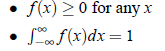Kurtosis:

Definition 7. Kurtosis refers to the extent to which unimodal frequency curve is peaked.

Definition 8. Kurtosis is a measure that refers to the peakedness of the top of the curve . Kurtosis gives the degree of flatness or peakedness in the region about the mode of a frequency distribution.

According to Croxton and Cowden,

Definition 9. A measure of kurtosis indicates the degree to which the curve of a frequency distribution is peaked or flat topped.

Definition 10. Kurtosis is the property of a frequency distribution which expresses its relative peakedness.

Karl Pearson in 1905 introduced the three types of curves on the basis of kurtosis:

Mesokurtic: If the concentration of frequency in the middle of the frequency distribution is normal, the curve is known as mesokurtic.

Leptokurtic: If the frequencies are densely concentrated in the middle of the series, the will be more peaked than normal and is known as Leptokurtic.

Platykurtic: If the frequencies are not densely concentrated in the middle of the series, the curve will be more flat than normal and is known as platykurtic.

Measure of Kurtosis:

The measure of kurtosis based on central moments are given by Karl Pearson: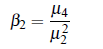• if β2 = 3, the curve is Mesokurtic or normal
• if β2 > 3, the curve is Leptokurtic or more peaked
• if β2 < 3, the curve is Platykurtic or flat topped.

The measure of Kurtosis is also represented by gamma two,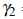= β2−3

• if= 0,the curve is Mesokurtic
• if> 0,the curve in Leptokurtic
• if< 0, the curve is Platykurtic.

Probability Mass Function (PMF):

If X is a discrete random variable with distinct values x1, x2, . . . ,xn for which X has positive probabilities p1, p2, . . . , pn, then the function f (x) defined as: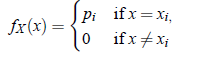is called the probability mass function of random variable X. Since P(S) = 1(Probability of sure event is one.), we must have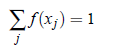Probability Density Function (PDF):

Similar to PMF, probability density function is defined for continuous random variable X. The funxtion f (x) defined as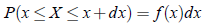such that,